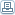# What Is Computer Programming Anyway?

 By: ShamsulComputer programming in plain language, is giving instructions to a computer to do something. Technically it is actually giving instructions to the microprocessor - the brain of a computer. If the instructions are only for the microprocessor, why a computer is so complicated with numerous types of hardware accessories? All the other hardware are to support the function of feeding the instructions to the microprocessor, and conveying the result from the microprocessor to its user - which can be a human or another computer or hardware. Every time you want a computer to do something you have to give the instructions. Luckily people are smart enough to figure out that since we might want the computer to repeat the same process over and over again, we better store the instructions into a permanent storage - hard disk, CD, flash memory etc. The stored instructions are called COMPUTER PROGRAM or computer software and the act of arranging the instructions is called COMPUTER PROGRAMMING and the person that is responsible to arrange the instructions is called .....COMPUTER PROGRAMMER ...do you see the pattern here? On the lowest level, a microprocessor only understands a limited set of instructions. To a microprocessor the instruction sets and data are read in “binary" form. Binary means 2 states – such as in on and off, high and low, left and right. To make it easier mathematically, binary normally is represented by 1 and 0. Electrically, 1 represents high voltage and 0 represents low voltage. On the hard disk, program instructions look just like a stream of 1s and 0s. But a microprocessor reads in the stream one chunk at a time. Among normal chunk sizes are 8, 16, and 32. Chunk size is normally referred to as instruction size. One binary data (that can be a 1 or a 0) is called a “bit". For example a data “1001" is a 4 bit data. Where first bit is 1, second bit is 0, third bit is another 0 and the fourth bit is 1. Bit is the computer terminology for “chunk".How instructions can be represented by bits?One bit data can only represent 1 out of 2 possible states – either 1 or 0. Which in real world can be used to represent things such as on or off, high or low, black or white – any 2 states condition? If we increase the instruction size to 2 bits, then we can represent 4 instructions – 00, or 01, or 10 or 11. If we increase the size to 3 bits then we can represent 8 possible instructions – 000, 001, 010, 011, 100, 101, 110 and 111 If you notice the trend from the above examples is that maximum possible number of instructions is the power of 2 of the bit size. That is 2 bits can represent maximum of 2^2 (which is 4) instructions, and 3 bits can represent maximum of 2^3 (which is 2x2x2 = 8) instructions. So 8 bits data can represent maximum of 2^8 (2x2x2x2x2x2x2x2 = 256) instructions (or states) and 32 bits data can represent 2^32 (4,294,967,296) instructions. You can actually read a program stream contents using certain editor – normally called HEX editor. Using these special text editors you can look at the instructions in binary, hexadecimal, octal, and decimal format. I’ll cover the details of what each of the above format (hex, oct and dec) means in other article. Permission is granted for this article to forward, reprint or distribute, use for ezine, website, offer as free bonus or part of a product for sales as long as no changes are made and the byline, copyright and the resource box is included.
 Programming